#A Beginner’s Guide To Understanding Convolutional Neural Networks Part 1

Interested in better understanding convolutional neural networks? Check out this first part of a very comprehensive overview of the topic.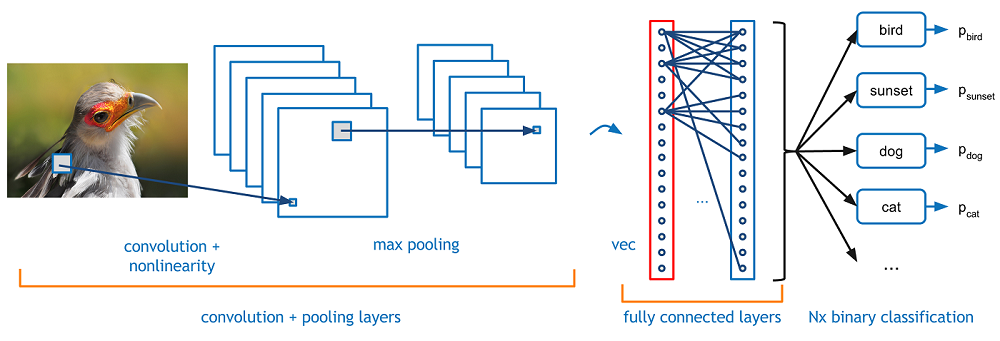### Introduction

Convolutional neural networks. Sounds like a weird combination of biology and math with a little CS sprinkled in, but these networks have been some of the most influential innovations in the field of computer vision. 2012 was the first year that neural nets grew to prominence as Alex Krizhevsky used them to win that year’s ImageNet competition (basically, the annual Olympics of computer vision), dropping the classification error record from 26% to 15%, an astounding improvement at the time.Ever since then, a host of companies have been using deep learning at the core of their services. Facebook uses neural nets for their automatic tagging algorithms, Google for their photo search, Amazon for their product recommendations, Pinterest for their home feed personalization, and Instagram for their search infrastructure.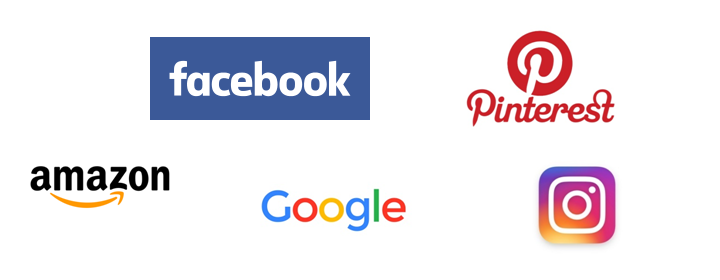However, the classic, and arguably most popular, use case of these networks is for image processing. Within image processing, let’s take a look at how to use these CNNs for image classification.

### The Problem Space

Image classification is the task of taking an input image and outputting a class (a cat, dog, etc) or a probability of classes that best describes the image. For humans, this task of recognition is one of the first skills we learn from the moment we are born and is one that comes naturally and effortlessly as adults. Without even thinking twice, we’re able to quickly and seamlessly identify the environment we are in as well as the objects that surround us. When we see an image or just when we look at the world around us, most of the time we are able to immediately characterize the scene and give each object a label, all without even consciously noticing. These skills of being able to quickly recognize patterns, generalize from prior knowledge, and adapt to different image environments are ones that we do not share with our fellow machines.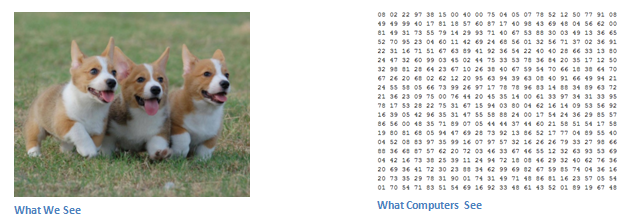### Inputs and Outputs

When a computer sees an image (takes an image as input), it will see an array of pixel values. Depending on the resolution and size of the image, it will see a 32 x 32 x 3 array of numbers (The 3 refers to RGB values). Just to drive home the point, let's say we have a color image in JPG form and its size is 480 x 480. The representative array will be 480 x 480 x 3. Each of these numbers is given a value from 0 to 255 which describes the pixel intensity at that point. These numbers, while meaningless to us when we perform image classification, are the only inputs available to the computer.  The idea is that you give the computer this array of numbers and it will output numbers that describe the probability of the image being a certain class (.80 for cat, .15 for dog, .05 for bird, etc).

### What We Want the Computer to Do

Now that we know the problem as well as the inputs and outputs, let’s think about how to approach this. What we want the computer to do is to be able to differentiate between all the images it’s given and figure out the unique features that make a dog a dog or that make a cat a cat. This is the process that goes on in our minds subconsciously as well. When we look at a picture of a dog, we can classify it as such if the picture has identifiable features such as paws or 4 legs. In a similar way, the computer is able perform image classification by looking for low level features such as edges and curves, and then building up to more abstract concepts through a series of convolutional layers. This is a general overview of what a CNN does. Let’s get into the specifics.

### Biological Connection

But first, a little background. When you first heard of the term convolutional neural networks, you may have thought of something related to neuroscience or biology, and you would be right. Sort of. CNNs do take a biological inspiration from the visual cortex. The visual cortex has small regions of cells that are sensitive to specific regions of the visual field. This idea was expanded upon by a fascinating experiment by Hubel and Wiesel in 1962 (Video) where they showed that some individual neuronal cells in the brain responded (or fired) only in the presence of edges of a certain orientation. For example, some neurons fired when exposed to vertical edges and some when shown horizontal or diagonal edges. Hubel and Wiesel found out that all of these neurons were organized in a columnar architecture and that together, they were able to produce visual perception. This idea of specialized components inside of a system having specific tasks (the neuronal cells in the visual cortex looking for specific characteristics) is one that machines use as well, and is the basis behind CNNs.

### Structure

Back to the specifics. A more detailed overview of what CNNs do would be that you take the image, pass it through a series of convolutional, nonlinear, pooling (downsampling), and fully connected layers, and get an output. As we said earlier, the output can be a single class or a probability of classes that best describes the image. Now, the hard part is understanding what each of these layers do. So let’s get into the most important one.

### First Layer – Math Part

The first layer in a CNN is always a Convolutional Layer. First thing to make sure you remember is what the input to this conv (I’ll be using that abbreviation a lot) layer is. Like we mentioned before, the input is a 32 x 32 x 3 array of pixel values. Now, the best way to explain a conv layer is to imagine a flashlight that is shining over the top left of the image. Let’s say that the light this flashlight shines covers a 5 x 5 area. And now, let’s imagine this flashlight sliding across all the areas of the input image. In machine learning terms, this flashlight is called a filter(or sometimes referred to as a neuron or a kernel) and the region that it is shining over is called the receptive field. Now this filter is also an array of numbers (the numbers are called weights orparameters). A very important note is that the depth of this filter has to be the same as the depth of the input (this makes sure that the math works out), so the dimensions of this filter is 5 x 5 x 3. Now, let’s take the first position the filter is in for example.  It would be the top left corner. As the filter is sliding, or convolving, around the input image, it is multiplying the values in the filter with the original pixel values of the image (aka computing element wise multiplications). These multiplications are all summed up (mathematically speaking, this would be 75 multiplications in total). So now you have a single number. Remember, this number is just representative of when the filter is at the top left of the image. Now, we repeat this process for every location on the input volume. (Next step would be moving the filter to the right by 1 unit, then right again by 1, and so on). Every unique location on the input volume produces a number. After sliding the filter over all the locations, you will find out that what you’re left with is a 28 x 28 x 1 array of numbers, which we call an activation map or feature map. The reason you get a 28 x 28 array is that there are 784 different locations that a 5 x 5 filter can fit on a 32 x 32 input image. These 784 numbers are mapped to a 28 x 28 array.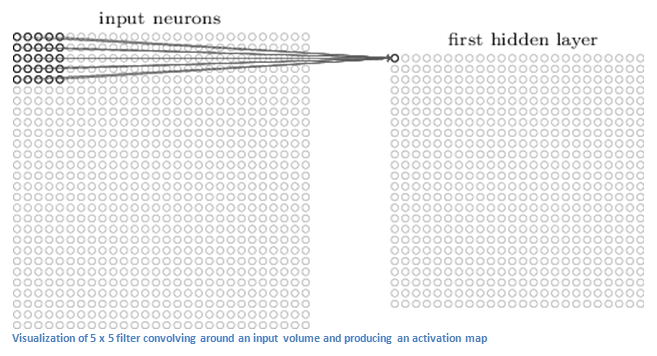(Quick Note: Some of the images, including the one above, I used came from this terrific book, "Neural Networks and Deep Learning" by Michael Nielsen. Strongly recommend.)

Let’s say now we use two 5 x 5 x 3 filters instead of one. Then our output volume would be 28 x 28 x 2. By using more filters, we are able to preserve the spatial dimensions better. Mathematically, this is what’s going on in a convolutional layer.

### First Layer – High Level Perspective

However, let’s talk about what this convolution is actually doing from a high level. Each of these filters can be thought of as feature identifiers. When I say features, I’m talking about things like straight edges, simple colors, and curves. Think about the simplest characteristics that all images have in common with each other. Let’s say our first filter is 7 x 7 x 3 and is going to be a curve detector. (In this section, let’s ignore the fact that the filter is 3 units deep and only consider the top depth slice of the filter and the image, for simplicity.)As a curve detector, the filter will have a pixel structure in which there will be higher numerical values along the area that is a shape of a curve (Remember, these filters that we’re talking about as just numbers!).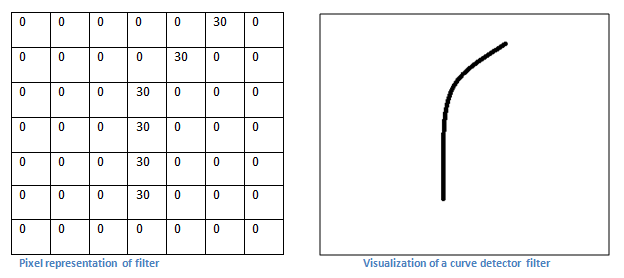Now, let’s go back to visualizing this mathematically. When we have this filter at the top left corner of the input volume, it is computing multiplications between the filter and pixel values at that region. Now let’s take an example of an image that we want to classify, and let’s put our filter at the top left corner.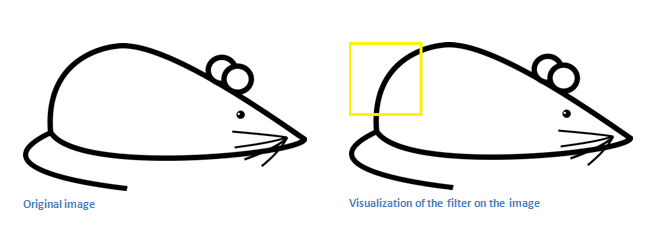Remember, what we have to do is multiply the values in the filter with the original pixel values of the image.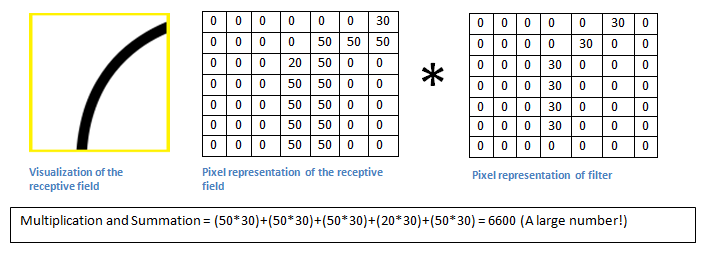Basically, in the input image, if there is a shape that generally resembles the curve that this filter is representing, then all of the multiplications summed together will result in a large value! Now let’s see what happens when we move our filter.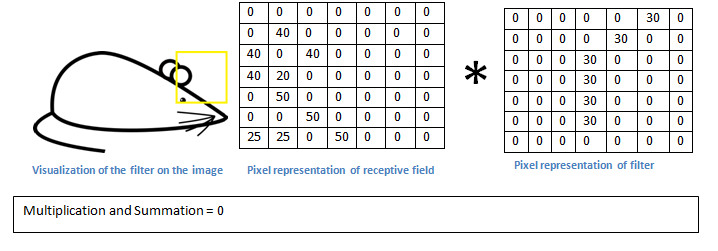The value is much lower! This is because there wasn’t anything in the image section that responded to the curve detector filter. Remember, the output of this conv layer is an activation map. So, in the simple case of a one filter convolution (and if that filter is a curve detector), the activation map will show the areas in which there at mostly likely to be curves in the picture. In this example, the top left value of our 28 x 28 x 1 activation map will be 6600. This high value means that it is likely that there is some sort of curve in the input volume that caused the filter to activate. The top right value in our activation map will be 0 because there wasn’t anything in the input volume that caused the filter to activate (or more simply said, there wasn’t a curve in that region of the original image). Remember, this is just for one filter. This is just a filter that is going to detect lines that curve outward and to the right. We can have other filters for lines that curve to the left or for straight edges. The more filters, the greater the depth of the activation map, and the more information we have about the input volume.

Disclaimer: The filter I described in this section was simplistic for the main purpose of describing the math that goes on during a convolution. In the picture below, you’ll see some examples of actual visualizations of the filters of the first conv layer of a trained network. Nonetheless, the main argument remains the same. The filters on the first layer convolve around the input image and “activate” (or compute high values) when the specific feature it is looking for is in the input volume.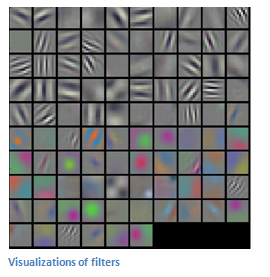(Quick Note: The above image came from Stanford's CS 231N course taught by Andrej Karpathy and Justin Johnson. Recommend for anyone looking for a deeper understanding of CNNs.)